• Python
• 机器学习
• 深度学习

# 前言

## 关于异常值和鲁棒的损失的问题：

::: hljs-center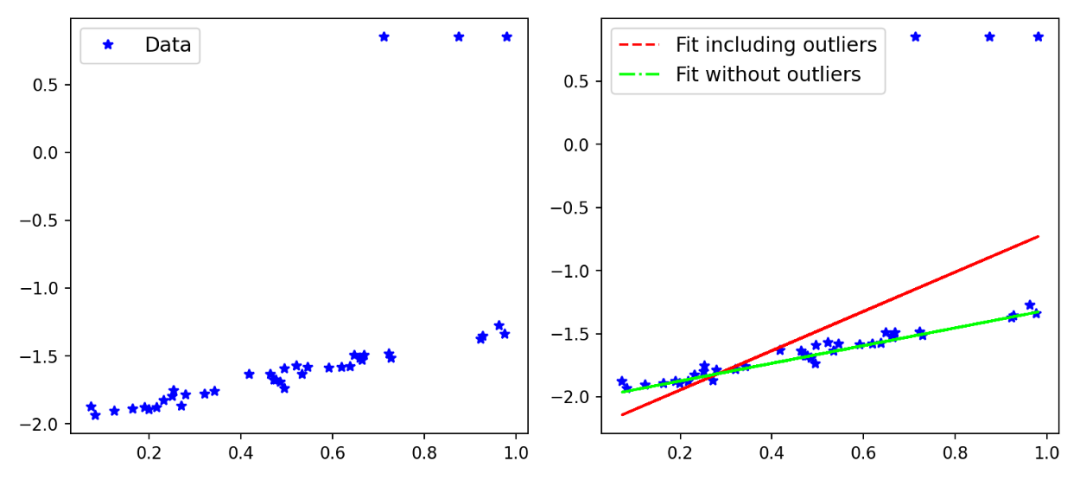:::
::: hljs-center

:::

## 鲁棒的以及自适应的损失：

::: hljs-center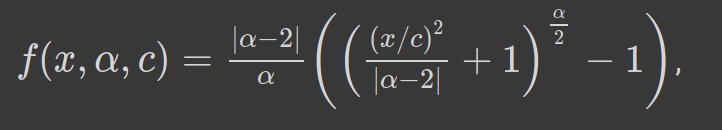:::

::: hljs-center

:::
α控制损失函数的鲁棒性。c可以看作是一个尺度参数，在x=0附近控制弯曲的尺度。由于α是超参数，我们可以看到，对于α的不同值，损失函数具有相似的形式。让我们看看下面：
::: hljs-center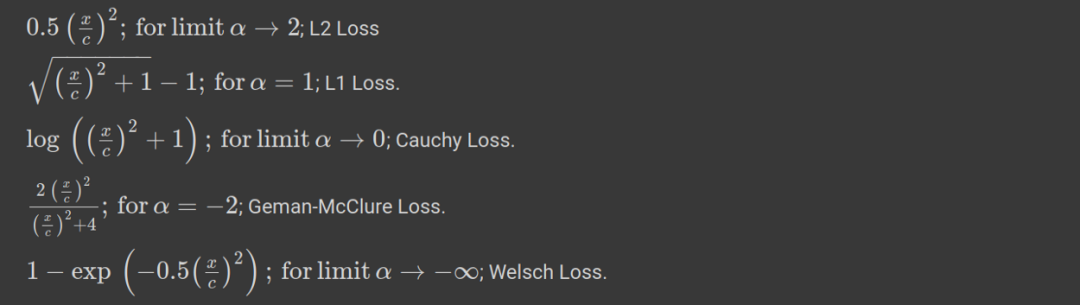:::
::: hljs-center

:::

::: hljs-center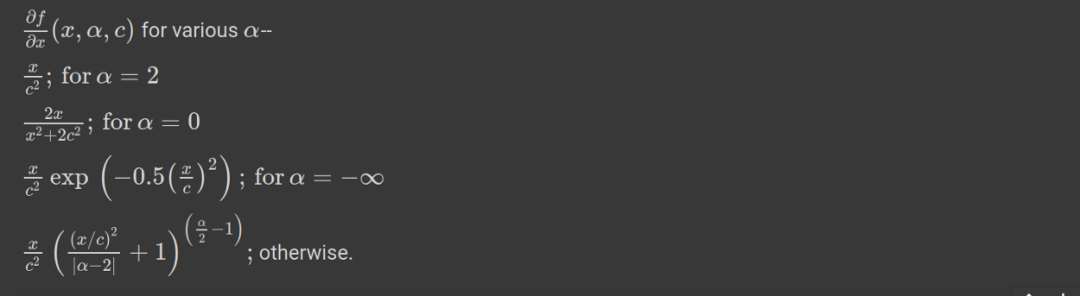:::
::: hljs-center

:::

## 自适应损失的表现极其导数：

1.损失函数对x, α和c>0是平滑的，适用于基于梯度的优化。
2.在原点处损失始终为零，当*|x|>0*时，单调增加。具有单调性损失也可以取对数损失进行比较。
3.损失相对于α也是单调递增的。这一特性对于损失函数的鲁棒性非常重要，因为我们可以从一个较高的α值开始，然后在优化过程中逐渐(平稳地)减少，从而使鲁棒估计避免局部极小值。
4.我们看到当|x|<c时，对于不同的α值，导数几乎是线性的。这意味着当它们很小的时候，导数与残差的大小成比例。
5.对于 α = 2，整个导数与残差的大小成比例。这是一般的MSE (L2)损失的性质。
6.对于α = 1(L1损失)，我们可以看到，在|x|>c之外，导数的幅度饱和到一个恒定值(恰好是1/c)。这意味着残差的影响永远不会超过一个固定的数值。
7.对于 α < 1导数的大小随|x|>c的变化而减小。这意味着随着残差的增加，它对梯度的影响较小，因此在梯度下降过程中，离群点的影响较小。

::: hljs-center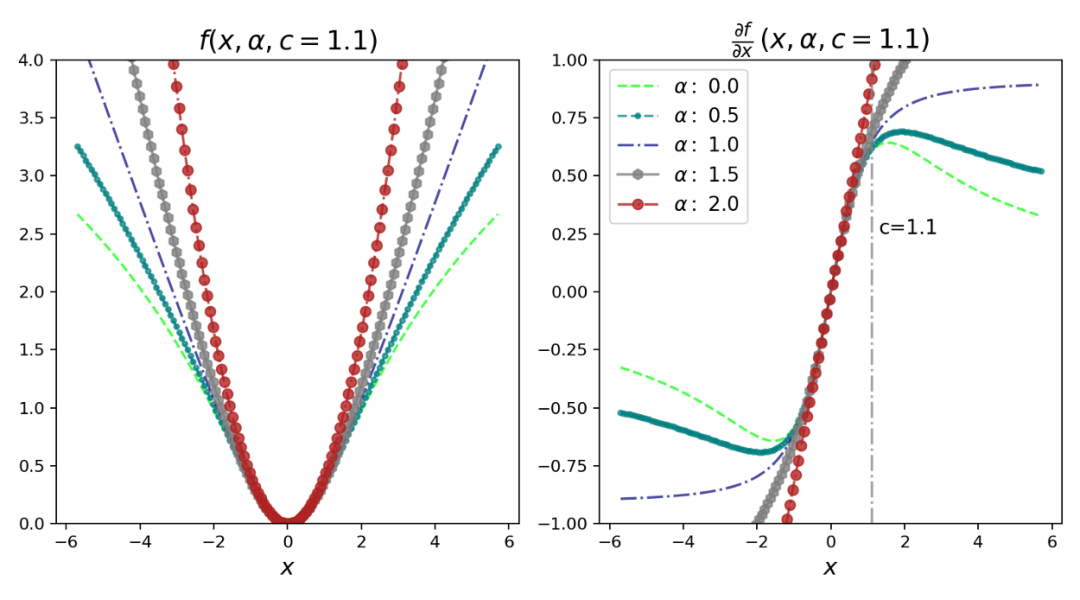:::

::: hljs-center

:::

::: hljs-center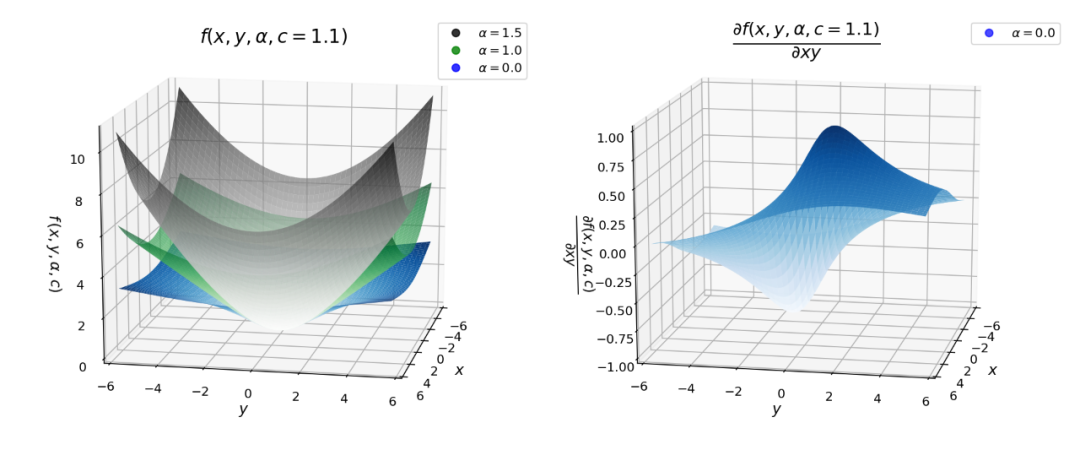:::

!pip install git+https://github.com/jonbarron/robust_loss_pytorchimport robust_loss_pytorch


import numpy as np
import torch

scale_true = 0.7
shift_true = 0.15

x = np.random.uniform(size=n)
y = scale_true * x + shift_true
y = y + np.random.normal(scale=0.025, size=n) # add noise

y = np.where(flip_mask, 0.05 + 0.4 * (1. — np.sign(y — 0.5)), y)
# include outliers

x = torch.Tensor(x)
y = torch.Tensor(y)


class RegressionModel(torch.nn.Module):

def __init__(self):
super(RegressionModel, self).__init__()
self.linear = torch.nn.Linear(1, 1)
## applies the linear transformation.

def forward(self, x):
return self.linear(x[:,None])[:,0] # returns the forward pass



regression = RegressionModel()
params = regression.parameters()
optimizer = torch.optim.Adam(params, lr = 0.01)

for epoch in range(2000):
y_i = regression(x)
# Use general loss to compute MSE, fixed alpha, fixed scale.
loss = torch.mean(robust_loss_pytorch.general.lossfun(
y_i — y, alpha=torch.Tensor([2.]), scale=torch.Tensor([0.1])))
loss.backward()
optimizer.step()



::: hljs-center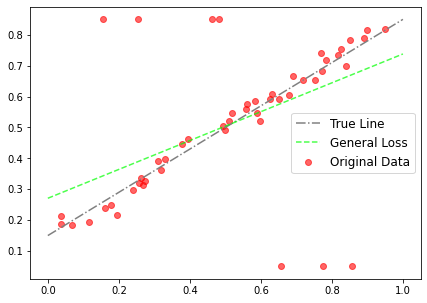:::

regression = RegressionModel()
num_dims = 1, float_dtype=np.float32)
optimizer = torch.optim.Adam(params, lr = 0.01)
for epoch in range(2000):
y_i = regression(x)
# (y_i - y)[:, None] # numpy array or tensor
loss.backward()
optimizer.step()



::: hljs-center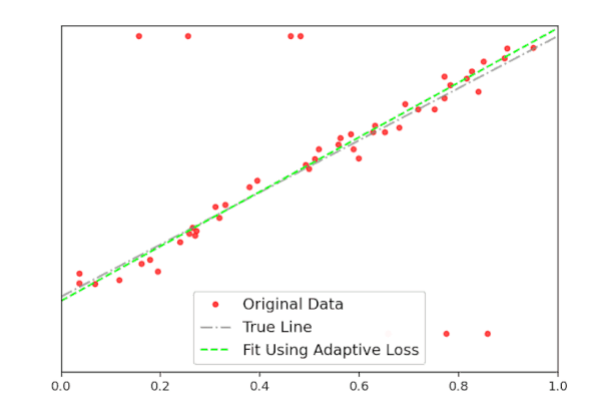:::

::: hljs-center

:::

## 引用:

 A General and Adaptive Robust Loss Function”; J. Barron, Google Research.
 Robust-Loss: Linear regression example; Jon Barron’s GitHub.
 Surface plot of robust loss and animation: GitHub Link.

2

1

1